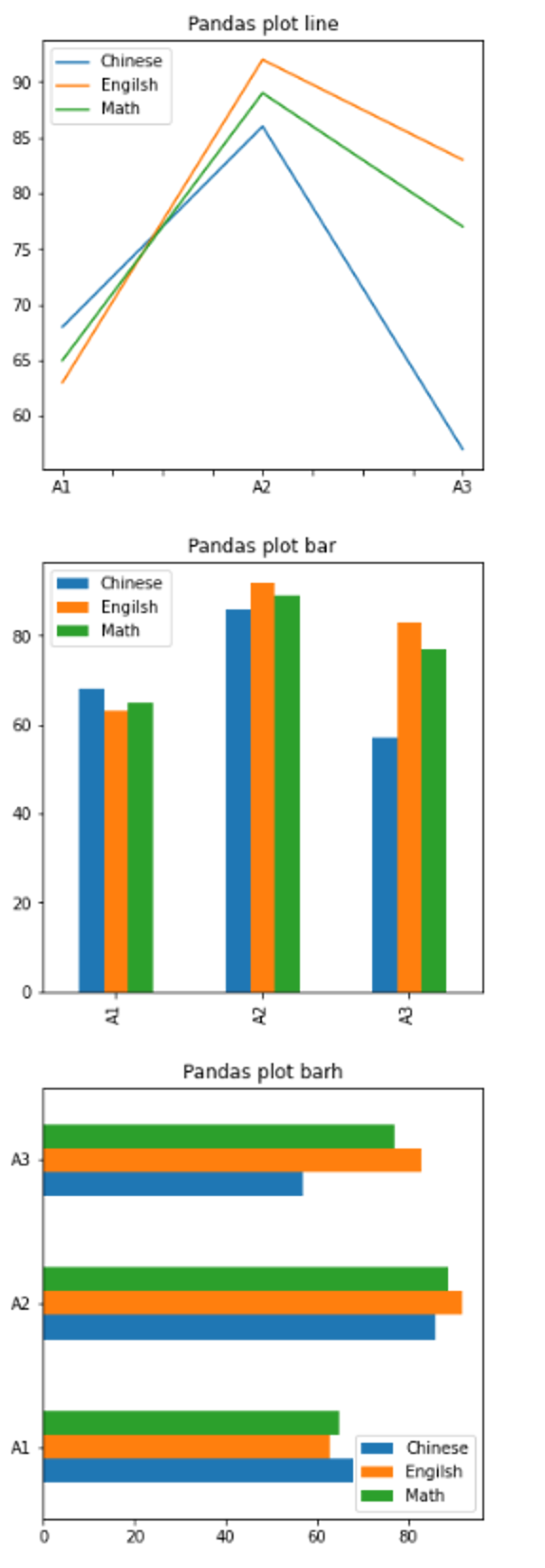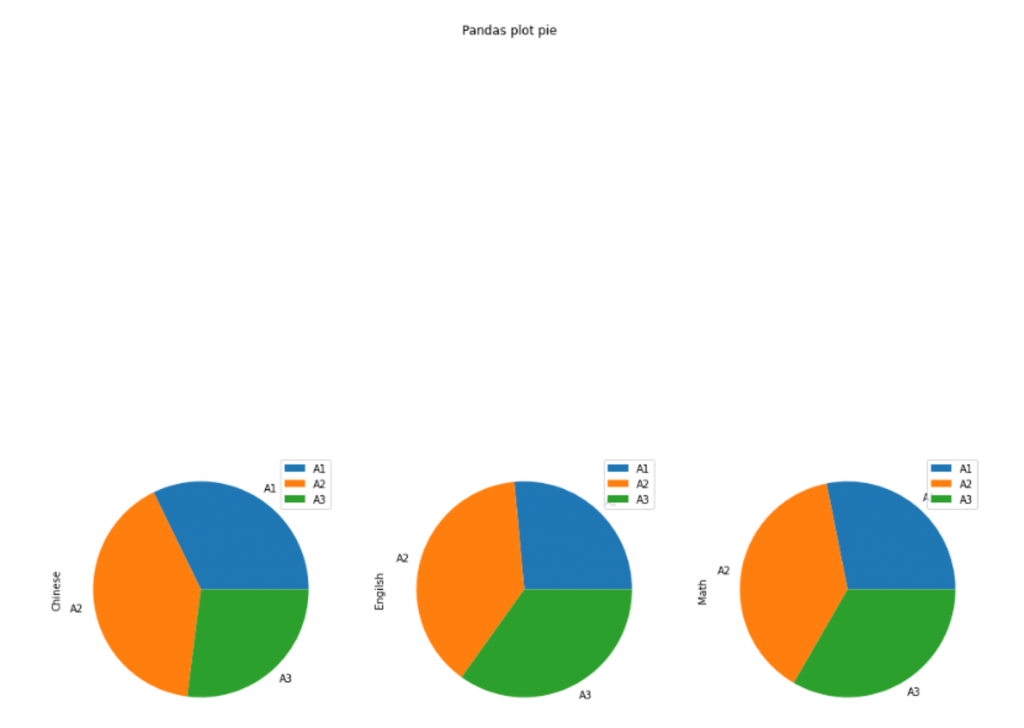#DAY 29
1
Software Development

## [2020鐵人賽Day29]糊裡糊塗Python就上手-Pandas的觀念與運用(下)

### DataFrame 資料操作

#### DataFrame 資料排序

df.sort_values(by = "欄位"[, ascending = 布林值]) 根據資料數值排序，by 作為排序值的欄位，ascending的話，True 表示遞增(未設定此參數的話，此為預設值))，False 表示遞減
df.sort_index(axis=軸向編號[, ascending = 布林值]) 根據軸向排序，axis 軸向編號，0 表示依照索引名稱排序，1 表示依照欄位名稱排序

``````import pandas as pd

dictValue = {
"Chinese": {"A1":68, "A2":86, "A3":57},
"Engilsh": {"A1":63, "A2":92, "A3":83},
"Math": {"A1":65, "A2":89, "A3":77}
}
df = pd.DataFrame(dictValue)
print(df) # 印出所有數值
print("-" * 40)

print("使用欄位「Math」做遞減排序:\n", df.sort_values(by="Math", ascending=False))
print("-" * 40)
print("使用索引名稱做遞減排序:\n", df.sort_index(axis=0, ascending=False))
``````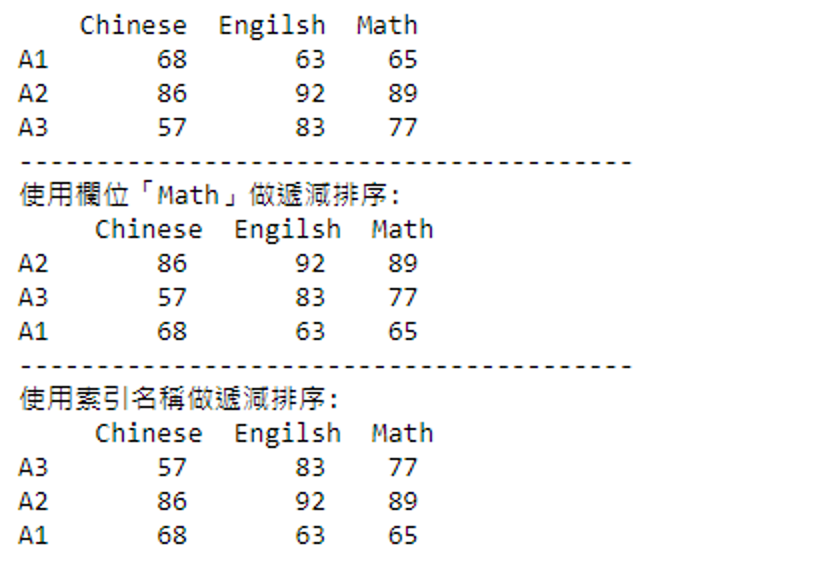#### DataFrame 資料更新

``````df.loc["索引名稱"]["欄位名稱"] = 將更改的數值
``````

``````import pandas as pd

dictValue = {
"Chinese": {"A1":68, "A2":86, "A3":57},
"Engilsh": {"A1":63, "A2":92, "A3":83},
"Math": {"A1":65, "A2":89, "A3":77}
}
df = pd.DataFrame(dictValue)
print(df) # 印出所有數值
print("-" * 40)

# 情境一
df.loc["A2"]["Engilsh"] = 76 # 將 A2 的 Engilsh 更改為 76

# 情境二
df.loc["A3", :] = 100 # 將 A3 的所有欄位更改為 100
print(df) # 印出所有數值
``````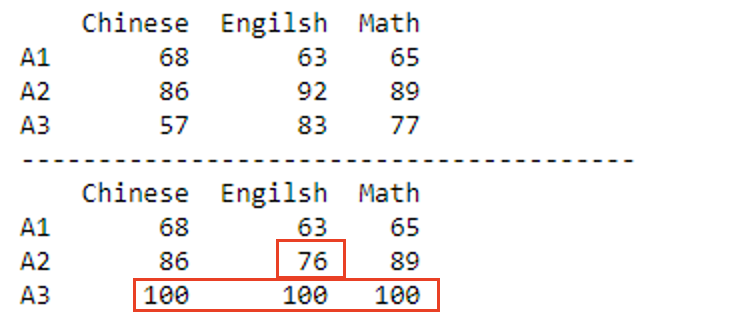#### DataFrame 資料刪除

(axis 軸向編號，0 代表為列(索引名稱)，1 代表為欄(欄位名稱))

``````變數名稱 = df.drop(索引名稱或欄位名稱[, axis=軸向編號])
``````

``````import pandas as pd

dictValue = {
"Chinese": {"A1":68, "A2":86, "A3":57},
"Engilsh": {"A1":63, "A2":92, "A3":83},
"Math": {"A1":65, "A2":89, "A3":77}
}
df = pd.DataFrame(dictValue)
print(df) # 印出所有數值
print("-" * 40)

# 情境一
df2 = df.drop("A3", axis=0) # 刪除索引 A3 所有數值
print(df2) # 印出所有數值
print("-" * 40)

# 情境二
df3 = df.drop("Engilsh", axis=1) # 刪除欄位 Engilsh 所有數值
print(df3) # 印出所有數值
``````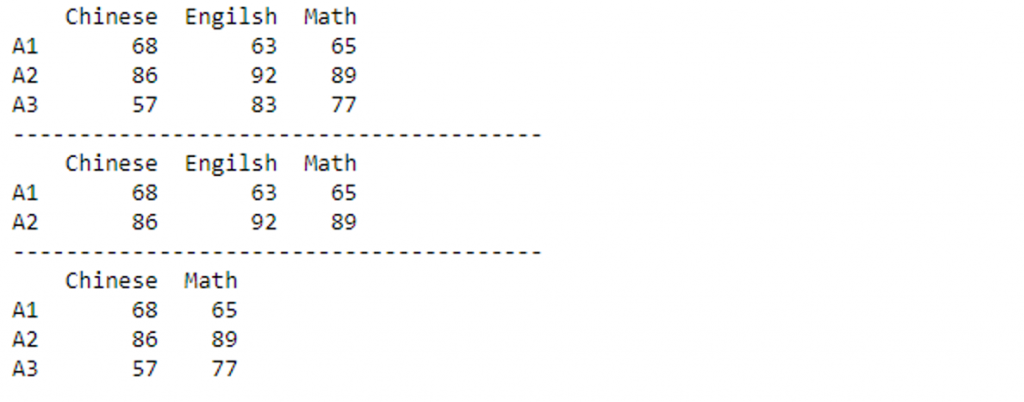``````# 依照給予的索引位置刪除，軸向編號 axis=0

# 依照給予的欄位位置刪除，軸向編號 axis=1

``````

### Pandas 資料存取

Pandas 可以從 CSV、Excel、SQL、JSON、HTML中取得資料，匯入後再轉換為 DataFrame 進行資料的修改、排序等等動作

#### Pandas 儲存資料

to_csv 儲存為 CSV 檔案資料
to_excel 儲存為 Excel 檔案資料
to_sql 儲存為 SQL 資料庫資料
to_json 儲存為 JSON 格式資料
to_html 儲存為 HTML 網頁表格資料

#### 實際體驗

to_csv 語法：

``````pandas.to_csv(檔案名稱[, header=布林值, index=布林值, encoding=編碼, sep=分隔符號])
``````

``````import pandas as pd

dictValue = {
"Chinese": {"A1":68, "A2":86, "A3":57},
"Engilsh": {"A1":63, "A2":92, "A3":83},
"Math": {"A1":65, "A2":89, "A3":77}
}
df = pd.DataFrame(dictValue)

df.to_csv('pandas_example.csv', encoding='utf-8-sig')
``````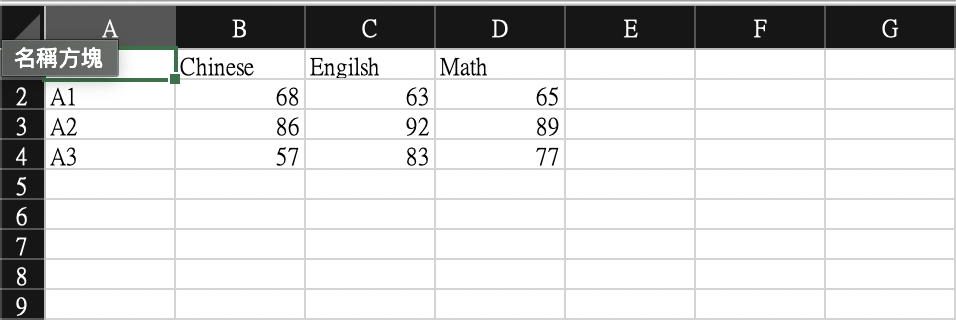``````pandas.read_csv(檔案名稱[, header=欄位列, index=索引列, encoding=編碼, sep=分隔符號])
``````

``````import pandas as pd

print(data)
print(type(data))
``````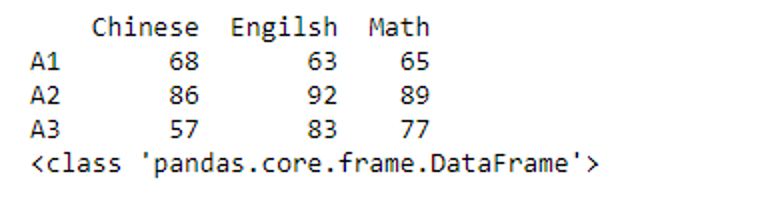### Pandas 視覺化呈現數據

#### plot 繪製

Pandas 模組是以 DataFrame資料的 plot() 方法將數據繪製出來，其語法為：
(可以不用設定其餘參數即可呈現，kind 預設為 line 折線圖)

``````DataFrame.plot([kind='圖形mode', title=None, figsize=None, fontsize=None, xticks=None, yticks=None, xlim=None, ylim=None, grid=False, legend=True])
``````
DataFrame plot參數 說明 預設值
kind 圖形模式 line
title 圖形標題 None
figsize 圖形大小 None
fontsize 圖形 x、y 軸的字體大小 None
xticks 圖形 x 軸的刻度值 None
yticks 圖形 y 軸的刻度值 None
xlim 圖形 x 軸的刻度範圍 None
ylim 圖形 y 軸的刻度範圍 None
grid 是否顯示隔線 False
legend 是否顯示圖示說明 True
kind 參數值 說明

line｜折線圖
bar｜長條圖
barh｜橫條圖
pie｜圓餅圖
hist｜直方圖

(這邊的「圓餅圖」需要使用到 subplot=True 這個參數，這會讓多張圖表放置在同一個區域中)

``````import pandas as pd

df.plot(kind='line', figsize=(5,5), title='Pandas plot line') # 折線圖
df.plot(kind='bar', figsize=(5,5), title='Pandas plot bar') # 長條圖
df.plot(kind='barh', figsize=(5,5), title='Pandas plot barh') # 橫條圖
df.plot(kind='pie', figsize=(16,16), title='Pandas plot pie', subplots=True) # 圓餅圖
``````